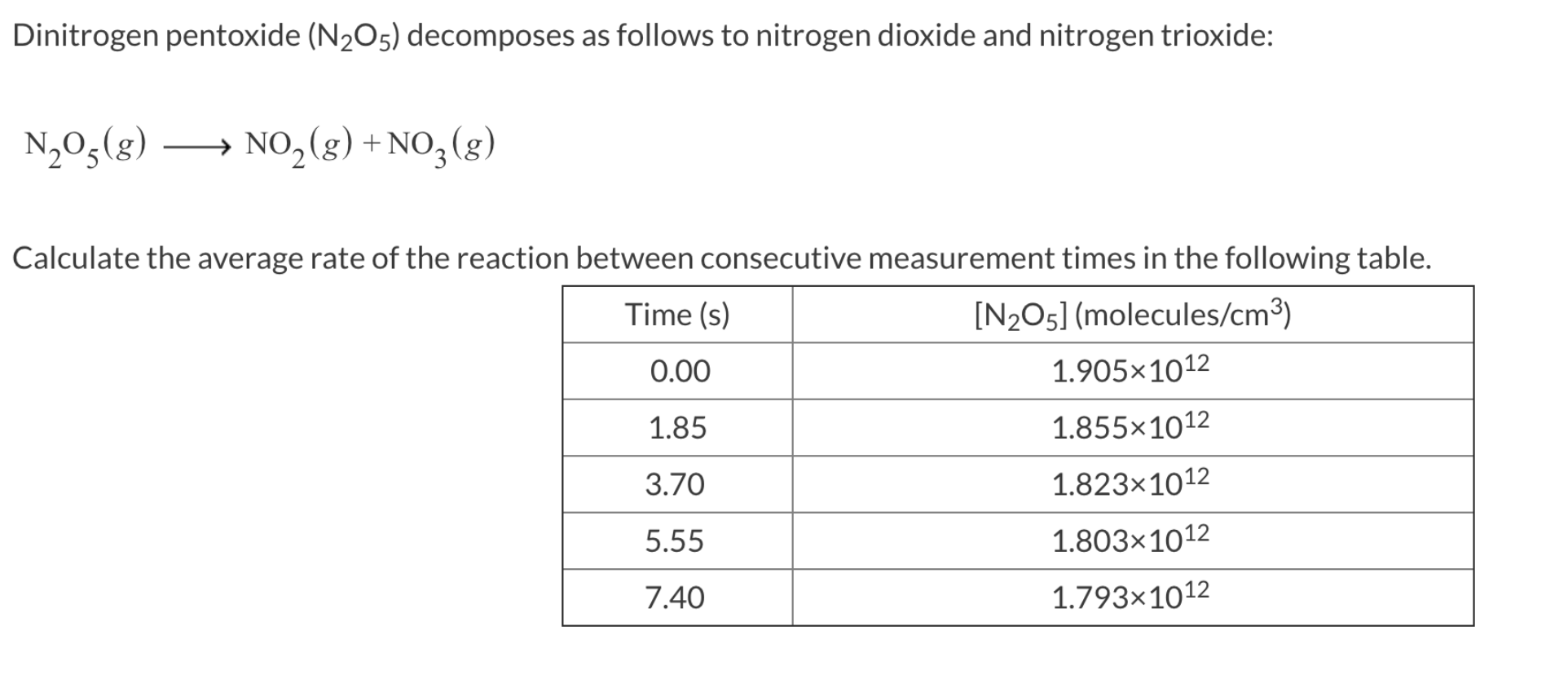Dinitrogen pentoxide (N2Os) decomposes as follows to nitrogen dioxide and nitrogen trioxide:N20,gNO2)+NO3(g)Calculate the average rate of the reaction between consecutive measurement times in the following table.[N205] (molecules/cm3)1.905x10121.855x10121.823x10121.803x10121.793x1012Time (s)0.001.855.557.40

Question

Express every answer to two significant figures.

Rate 1 =

Rate 2 =

Rate 3 =

Rate 4 =help_outlineImage TranscriptioncloseDinitrogen pentoxide (N2Os) decomposes as follows to nitrogen dioxide and nitrogen trioxide: N20,gNO2)+NO3(g) Calculate the average rate of the reaction between consecutive measurement times in the following table. [N205] (molecules/cm3) 1.905x1012 1.855x1012 1.823x1012 1.803x1012 1.793x1012 Time (s) 0.00 1.85 5.55 7.40 fullscreen
Step 1

Rate of a particular reaction is the concentration change of reactant or product divided by change in time. The rate of the reaction can be determined as below.

Step 2

Rate 1 can be determined as given.

Step 3

Rate 2 can be determi...

Want to see the full answer?

See Solution

Want to see this answer and more?

Our solutions are written by experts, many with advanced degrees, and available 24/7

See Solution
Tagged in

Chemistry